Courses

# Channel Capacity Electronics and Communication Engineering (ECE) Notes | EduRev

## Communication Theory

Created by: Machine Experts

## Electronics and Communication Engineering (ECE) : Channel Capacity Electronics and Communication Engineering (ECE) Notes | EduRev

The document Channel Capacity Electronics and Communication Engineering (ECE) Notes | EduRev is a part of the Electronics and Communication Engineering (ECE) Course Communication Theory.
All you need of Electronics and Communication Engineering (ECE) at this link: Electronics and Communication Engineering (ECE)

CHANNEL CAPACITY:

In electrical engineering, computer science and information theory, channel capacity is the tightest upper bound on the amount of information that can be reliably transmitted over a communications channel. By the noisychannel coding theorem, the channel capacity of a given channel is the limiting information rate (in units of information per unit time) that can be achieved with arbitrarily small error probability.

Information theory, developed by Claude E. Shannon during World War II, defines the notion of channel capacity and provides a mathematical model by which one can compute it. The key result states that the capacity of the channel, as defined above, is given by the maximum of the mutual information between the input and output of the channel, where the maximization is with respect to the input distribution

Formal definition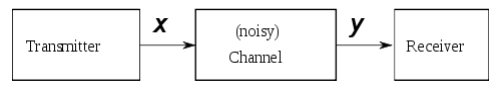Let X represent the space of signals that can be transmitted, and Y the space of signals received, during a block of time over the channel.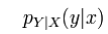Let be the conditional distribution function of Y given X. Treating the channel as a known statistic system, pY | X(y | x) is an inherent fixed property of the communications channel (representing the nature of the noise in it). Then the joint distribution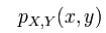of X and Y is completely determined by the channel and by the choice of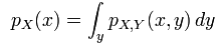Under these constraints, next maximize the amount of information, or the message, that one can communicate over the channel. The appropriate measure for this is the mutual information I(X;Y), and this maximum mutual information is called the channel capacity and is given by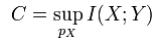Noisy-channel coding theorem:

The noisy-channel coding theorem states that for any ε > 0 and for any rate R less than the channel capacity C, there is an encoding and decoding scheme that can be used to ensure that the probability of block error is less than ε for a sufficiently long code. Also, for any rate greater than the channel capacity, the probability of block error at the receiver goes to one as the block length goes to infinity.

Example application:

An application of the channel capacity concept to an additive white Gaussian noise (AWGN) channel with B Hz bandwidth and signal-to-noise ratio S/N is the Shannon–Hartley theorem: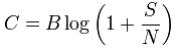C is measured in bits per second if the logarithm is taken in base 2, or nats per second if the natural logarithm is used, assuming B is in hertz; the signal and noise powers S and N are measured in watts or volts2 , so the signal-tonoise ratio here is expressed as a power ratio, not in decibels (dB); since figures are often cited in dB, a conversion may be needed. For example, 30 dB is a power ratio of 1030 / 10 = 103 = 1000.

Slow-fading channel: In a slow-fading channel, where the coherence time is greater than the latency requirement, there is no definite capacity as the maximum rate of reliable communications supported by the channel, log2(1 + | h |2 SNR), depends on the random channel gain | h |2 . If the transmitter encodes data at rate R [bits/s/Hz], there is a certain probability that the decoding error probability cannot be made arbitrarily small,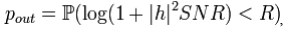in which case the system is said to be in outage. With a non-zero probability that the channel is in deep fade, the capacity of the slow-fading channel in strict sense is zero. However, it is possible to determine the largest value of R such that the outage probability pout is less than ε. This value is known as the ε-outage capacity.

In a fast-fading channel, where the latency requirement is greater than the coherence time and the codeword length spans many coherence periods, one can average over many independent channel fades by coding over a large number of coherence time intervals. Thus, it is possible to achieve a reliable rate of communication of Elog2(1 + | h |2 SNR)) [bits/s/Hz] and it is meaningful to speak of this value as the capacity of the fast-fading channel.

RATE DISTORTION THEORY:

Rate–distortion theory is a major branch of information theory which provides the theoretical foundations for lossy data compression; it addresses the problem of determining the minimal amount of entropy (or information) R that should be communicated over a channel, so that the source (input signal) can be approximately reconstructed at the receiver (output signal) without exceeding a given distortion D.

Offer running on EduRev: Apply code STAYHOME200 to get INR 200 off on our premium plan EduRev Infinity!

,

,

,

,

,

,

,

,

,

,

,

,

,

,

,

,

,

,

,

,

,

;